Our first bound is a recursive upper bound on the covering radius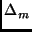of the m-th code of an arbitrary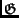-family. We shall use the termto refer to the corresponding term in Definition 1 for this m-th code.

Lemma 1   For any-family of codes and m > 1,Proof: Consider constructing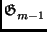from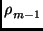using Theorem 3. Any two coset leaders l and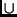(l ) ofmust each have weight at most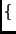, by the definition of the covering radius. Consider the weight of a corresponding coset leader of, based on Theorem 3:

 minwt(a | l ), wt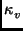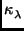|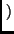(l )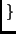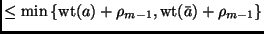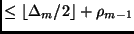for all a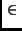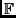2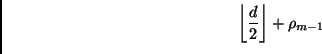. Since the-construction implicitly demands that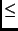d, we may conclude that any coset leader ofmust have weight no greater thanwhich bounds the covering radius ofand proves the lemma.

width4pt depth2pt height6pt

With some combinatorial analysis, we can also establish a recursive lower bound on the covering radius of-codes. Specifically, we shall make use of the following simple combinatorial lemma, which we provide without proof.

Lemma 2   If a > 3b > 0 then

 a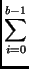>a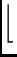. (10)

Theorem 4   For any-family of codes whose seed code has lengthit necessarily follows thatProof: Following convention, we let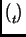denote the number of errors that the codes of a-family can correct. It is well-known that each vector in2nm - 1 of weightt must be a unique coset leader for. Moreover, using our assumption about n0 (which also holds for nm, since nmn0), Lemma 2 implies that

 nm - 1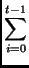>nm - 1. (11)

The left-hand side of (14) is the number of vectors of weight t, while the right-hand side is the number of vectors of weight < t. Thus, by the pigeon-hole principle, there must be at least one coset leader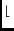of weight t whose companion(l ) has weight at least t. Sinced -for any-code, we may chooseto get: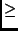min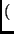wt(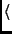a | l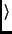), wt|(l )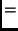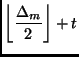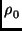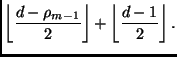width4pt depth2pt height6pt

The result in Theorem 4 also applies to all-families trivially seeded with the coded.

Lemma 3   For any trivially seeded-family of codes,If a-family is trivially seeded, then, necessarily, n0 = d and. Thus,so that Theorem 4 applies to the remaining codes in the family.

We now turn our attention to generating mappings which produce codes whose information rate is locally maximized. More specifically, for any code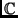with covering radius, we will consider only generating mappings f with the property that the Hamming distance fromtois exactly. We will call such mappings minimal generating mappings, and the corresponding family of codes minimal-codes, because they locally minimize length (and hence locally maximize information rate) for a given dimension and minimum-distance.

As an example, the generating mappings for the traditional lexicodes and the trellis-oriented lexicodes are both minimal. We may now easily strengthen Lemma 1 by observing in its proof that= d -for minimal-codes.

Corollary 1   For any minimal-family of codes,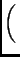The covering radius bounds we have developed for-codes translate naturally to length bounds.